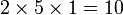# Groups of order 540

## Contents

See pages on algebraic structures of order 540| See pages on groups of a particular order

## Statistics at a glance

The order 540 has prime factorization:$\! 540 = 2^2 \cdot 3^3 \cdot 5^1 = 4 \cdot 27 \cdot 5$

Quantity Value Explanation
Total number of groups up to isomorphism 119
Total number of abelian groups up to isomorphism 6 (number of abelian groups of order$2^2$)$\times$ (number of abelian groups of order$3^3$)$\times$ (number of abelian groups of order$5^1$) = (number of unordered integer partitions of 2)$\times$ (number of unordered integer partitions of 3)$\times$ (number of unordered integer partitions of 1) =$2 \times 3 \times 1 = 6$. See classification of finite abelian groups and structure theorem for finitely generated abelian groups.
Total number of nilpotent groups up to isomorphism 10 (number of groups of order 4)$\times$ (number of groups of order 27)$\times$ (number of groups of order 5) =$2 \times 5 \times 1 = 10$.
Total number of solvable groups up to isomorphism 117 The only two non-solvable groups are direct product of A5 and Z9 and direct product of A5 and E9.
Number of simple non-abelian groups up to isomorphism 0

## GAP implementation

The order 540 is part of GAP's SmallGroup library. Hence, any group of order 540 can be constructed using the SmallGroup function by specifying its group ID. Also, IdGroup is available, so the group ID of any group of this order can be queried.

Further, the collection of all groups of order 540 can be accessed as a list using GAP's AllSmallGroups function.

Here is GAP's summary information about how it stores groups of this order, accessed using GAP's SmallGroupsInformation function:

gap> SmallGroupsInformation(540);

There are 119 groups of order 540.
They are sorted by their Frattini factors.
1 has Frattini factor [ 30, 1 ].
2 has Frattini factor [ 30, 2 ].
3 has Frattini factor [ 30, 3 ].
4 has Frattini factor [ 30, 4 ].
5 has Frattini factor [ 60, 6 ].
6 has Frattini factor [ 60, 7 ].
7 has Frattini factor [ 60, 8 ].
8 has Frattini factor [ 60, 9 ].
9 has Frattini factor [ 60, 10 ].
10 has Frattini factor [ 60, 11 ].
11 has Frattini factor [ 60, 12 ].
12 has Frattini factor [ 60, 13 ].
13 - 15 have Frattini factor [ 90, 5 ].
16 - 19 have Frattini factor [ 90, 6 ].
20 - 23 have Frattini factor [ 90, 7 ].
24 - 25 have Frattini factor [ 90, 8 ].
26 - 27 have Frattini factor [ 90, 9 ].
28 - 30 have Frattini factor [ 90, 10 ].
31 has Frattini factor [ 180, 19 ].
32 - 34 have Frattini factor [ 180, 20 ].
35 - 38 have Frattini factor [ 180, 21 ].
39 - 40 have Frattini factor [ 180, 22 ].
41 has Frattini factor [ 180, 23 ].
42 has Frattini factor [ 180, 24 ].
43 has Frattini factor [ 180, 25 ].
44 - 47 have Frattini factor [ 180, 26 ].
48 - 49 have Frattini factor [ 180, 27 ].
50 - 51 have Frattini factor [ 180, 28 ].
52 - 54 have Frattini factor [ 180, 29 ].
55 - 56 have Frattini factor [ 180, 30 ].
57 - 61 have Frattini factor [ 180, 31 ].
62 - 64 have Frattini factor [ 180, 32 ].
65 - 68 have Frattini factor [ 180, 33 ].
69 - 72 have Frattini factor [ 180, 34 ].
73 - 74 have Frattini factor [ 180, 35 ].
75 - 76 have Frattini factor [ 180, 36 ].
77 - 79 have Frattini factor [ 180, 37 ].
80 has Frattini factor [ 270, 23 ].
81 has Frattini factor [ 270, 24 ].
82 has Frattini factor [ 270, 25 ].
83 has Frattini factor [ 270, 26 ].
84 has Frattini factor [ 270, 27 ].
85 has Frattini factor [ 270, 28 ].
86 has Frattini factor [ 270, 29 ].
87 has Frattini factor [ 270, 30 ].
88 - 119 have trivial Frattini subgroup.

For the selection functions the values of the following attributes
are precomputed and stored:
IsAbelian, IsNilpotentGroup, IsSupersolvableGroup, IsSolvableGroup,
LGLength, FrattinifactorSize and FrattinifactorId.

This size belongs to layer 2 of the SmallGroups library.
IdSmallGroup is available for this size.